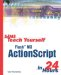# Comparisons and Operations

 I l @ ve RuBoard

Comparing two things in ActionScript is simple. You use standard mathematical symbols, such as = , < , and > .

#### Are the Values the Same?

You have already seen how the = symbol is used to assign a value to a variable. To differentiate between times when you want to assign a value and times when you want to compare two values, the double equals symbol, == , is used when you want to compare two things. The single equals operator is used to assign values to variables .

So, if you want to see whether variable a is the number 7, use == . Here is an example that places the results in the Output window:

` var a = 7; trace(a == 7); `

This code assigns the value 7 to the variable a by using the single equals symbols. It then compares a with 7 by using the double equals symbol.

When you test this code, the Output window will show "true." If you set a to 8 instead, the Output window will show "false" because 7 is not equal to 8.It is a common mistake, even for experts, to accidentally use a = in the place of a == . This can lead to a bug that is difficult to find because the difference can easily be missed by the eye. Watch out for this.

You can also use the == comparison to compare two strings. The following code compares a variable that contains a string with another string:

` var myString = "Hello World."; trace(myString == "Hello World."); trace(myString == "hello world."); `

When you run this program, you get both a "true" and a "false." This is because the first comparison matches the variable to exactly the same string, whereas the second comparison demonstrates that comparisons of strings take case into account.

Suppose that you want to test to see whether two values are not equal to each other. In this case, you use the special operator != , which just means "not equals":

` var a = 7; trace(a != 9); trace(a != 7); `

The first trace statement produces a "true" because a is not equal to 9. The second trace statement produces a false because a is indeed equal to 7, but we are trying to test for it not to be 7.

#### Less Than or Greater Than

You can also compare two things to see whether they are less than or greater than each other. To do this, use the standard mathematical symbols < and > . Here is an example:

` var a = 7; trace(a < 8); trace(a > 6); trace(a < 1); `

You should get a "true," "true," and "false" from this program. The variable a contains 7, which is less than 8, greater than 6, but is certainly not less than 1.

You can also use the <= or >= comparisons = (greater than or equal to) operator>=) operator>to find out whether a number is less than or equal to, or greater than or equal to, another number. Here is an example:

` var a = 7; trace(a <= 9); trace(a >= 5); trace(a >= 7); `

All three of the preceding statements are "true."

#### Operators

You can also modify the values of variables with operations. They are also standard mathematical symbols such as + and - for addition and subtraction. For multiplication, we use the * symbol. For division, we use the / symbol.

For instance, to add 4 to a variable that contains the number 7, we just use a second assignment statement that sets the value of the variable to its current value, plus 4:

` var a = 7; a = a + 4; trace(a); `

The result is 11, of course. ActionScript actually has some shorthand for performing the same addition. The += operation takes the current variable and adds the next number to it. Here is some code that does exactly the same thing as the previous code:

` var a = 7; a += 4; trace(a); `

There is another piece of shorthand that you should be familiar with. The ++ operator is like the += operator, but it adds exactly 1 to the number. Here is an example:

` var a = 7; a++; trace(a); `

The result is 8. Now try this:

` var a = 7; trace(a++); trace(a); `

The result is first a 7 and then an 8. What happened here? Well, the first trace command placed the current value of a in the Output window. Then the ++ operator added one to a . The second trace statement placed the new value in the Output window.

Now try this:

` var a = 7; trace(++a); trace(a); `

You will get two 8s this time. This is because when you place the ++ operator before the variable, the addition is performed before the command.

You can use -- as well as ++ to subtract rather than add. You can also use -= to subtract a number and *= and /= to multiply or divide a variable by a number.

 I l @ ve RuBoardSams Teach Yourself Flash MX ActionScript in 24 Hours
ISBN: 0672323850
EAN: 2147483647
Year: 2002
Pages: 272

flylib.com © 2008-2017.
If you may any questions please contact us: flylib@qtcs.net